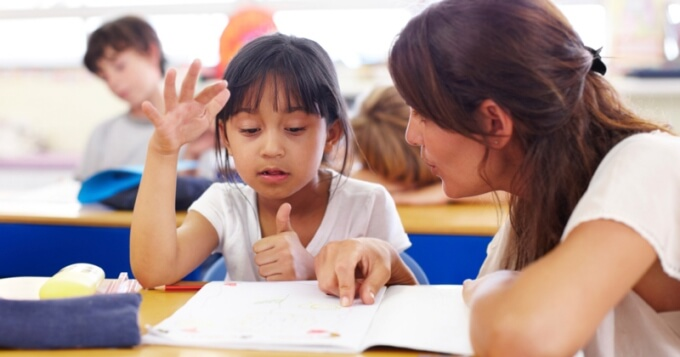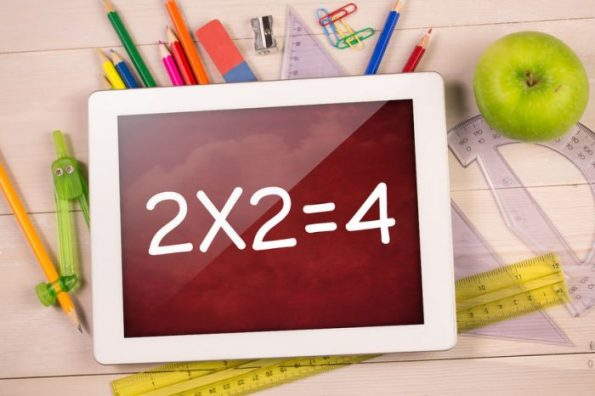# Teach the multiplication tables to primary school children

One of the first mathematical skills that children learn in school, once they know how to add and subtract, is to multiply. Multiplication is just a shortcut to take large sums more quickly, which means that it does not demand much memory but a good deal of reasoning. However, as the most usual is to ask the children to memorize the multiplication tables, they come to hate them. Luckily, there are other methods for children to learn to multiply.### Bring children to multiplication in five steps

#### 1. Wait for the right moment

One of the most frequent mistakes made by parents in the education of their children is to teach them content for which they are not yet prepared. Therefore, the first step to teach the multiplication tables, and probably one of the most important to not set a negative precedent, is to determine the appropriate time. The child will be prepared when he is mature enough to take on new content and has acquired essential knowledge such as addition and subtraction. Another factor that we often forget but that is essential is motivation, it is essential that the child is motivated by learning to multiply.

#### 2. Explain them what multiplication consists

Most children like to understand the reason for things, they are passionate about discovering what they are for, and in this way they are motivated. That is why it is essential that, rather than give the tables to learn from memory, you explain that multiplication is not more than the successive sum of the same number and is very useful to get quick accounts. Only when the child understands the usefulness of multiplication can he make sense of learning the tables.#### 3. Teach them the basic multiplications

Often parents, and even some teachers, think that learning to multiply is equivalent to memorizing but it is a serious mistake. Actually the tables are the last thing you should learn. First you must start with basic and very simple multiplications, such as 2X2, 2X3 or 3X3. It is about developing the ability to multiply and understand the process that is at the base. To work on the concept of multiplication, you must indicate that it is the successive sum of the same number, so that 2X3 means 2 times 3; that is: 3 + 3. And so on.

#### 4. Motivate to practice

Multiplication is not only logical, it also demands a good dose of practice, so the child will reinforce the knowledge acquired and, at the same time, will increase confidence in his abilities. Any place is good to practice, especially outside the home, so you understand the importance of this new skill. For example, when you go shopping you can ask: How much should I pay for 3 jars of jam if each one costs 2 euros?

#### 5. Go to the multiplication table

Once the child has understood how basic multiplications work, the time has come for you to show him the tables. You can use traditional tables or a custom one. At first the most important thing is for the child to manipulate and visualize the table so that he can understand it. Then you can go on to learn them, although you will have to gather memory, there are different tricks that will allow you to move faster in learning, such as:

• Table of 0. All numbers multiplied by 0 results in 0.
• Table of 1. All numbers multiplied by 1 result in the same number.
• Table of 2. Any number multiplied by 2 is twice the number.
• Table of 5. Numbers multiplied by 5 ends in 0 or 5, going in series that add 5 each time.
• Table of 10. It is only necessary to add a 0 to the multiplied number.